# AdvDCSM System Tool and TURBO CODING

NOTE: This section is Under-Edit if necessary: Construction began on February 8, 2014 and was finished on February 12, 2014.

This section's paper ('Turbo Coded Signaling over a Coherent Memoryless Channel') was revised on April 2, 2014 to add the work on Turbo Coding and M > 2 Modulation Schemes to the work on Turbo Coding and a Binary Modulation Scheme.

TURBO CODED SIGNALING over a Coherent Memoryless Channel

TURBO CODED SIGNALING over a COHERENT MEMORYLESS CHANNEL: M-ary Modulation Schemes and the Iterative Turbo Decoder

ADVANCED TURBO CODING and RATE MATCHING

TURBO CODED SIGNALING over a COHERENT MEMORYLESS CHANNEL: Rate Matching and the Iterative Turbo Decoder

ADVANCED TURBO DECODING and ITERATIVE STOPPING RULE

TURBO CODED SIGNALING over a COHERENT MEMORYLESS CHANNEL: Iterative Turbo Decoder and Stopping Rule

TURBO CODED SIGNALING over a MEMORY CHANNEL Part I

TURBO CODED SIGNALING over a MEMORY CHANNEL: M-ary Modulation Schemes, Rayleigh Fading, and Iterative Turbo Decoder

TURBO CODED SIGNALING over a MEMORY CHANNEL Part II

TURBO CODED SIGNALING over a MEMORY CHANNEL: Rate Matching, Rayleigh Fading, and the Iterative Turbo Decoder

Turbo Coded Signaling over a Coherent Memoryless Channel: M-ary Modulation Schemes and the Iterative Turbo Decoder

```by Darrell A. Nolta
March 26, 2014
```

The AdvDCSMT1DCSS Professional (T1 Version 2) system tool provides the capability to model and simulate Multiple Iteration Soft Input/Soft-Decision Output (SISO) Turbo Code (TC) Channel Decoding using a Turbo Decoding Algorithm where two SISO 'symbol-by-symbol' Maximum a Posteriori Probability (MAP) Algorithm component decoders (DEC1 & DEC2) are serial concatenated with a feedback loop. This decoding algorithm can be used in the cases for Turbo Coded Signaling over Memoryless Channels (MLC) with Additive White Gaussian Noise (AWGN).

Three MAP Decoding Algorithms (Max-Log-MAP, Log-MAP, and MAP) are supported by T1.

Note that an Information Bit Interleaver is used in the Turbo Encoder. And its corresponding SISO Interleaver and DeInterleaver are used in the Turbo Decoder.

T1 V2 provides the User the ability to select a Block, Pseudo-Random, Quadratic Permutation Polynomial, or Identity Interleaver type.

Also, this capability of T1 Professional supports decoding of TC Signaling over a BSC MLC, too.

In addition, T1 Professional provides the capability to model and simulate a Non-Iterative (Single Iteration) SISO TC Channel Decoding where DEC1 and DEC2 are serial concatenated without the feedback loop.

And this new feature provides the capability to puncture the Turbo Code so that the Turbo Code Rate is increased. One can achieve a Punctured Rate Rp of 1/2 for a parent TC Rate of 1/3; Rp of 1/2, 1/3, or 1/4 for a parent TC Rate of 1/5; and Rp of 1/2, 1/3, 1/4, 1/5, or 1/6 for a parent TC Rate of 1/7.

The incorporation of the Turbo Coded or Punctured Turbo Coded M-ary Signaling over an AWGN MLC feature into T1 V2 supports Binary Modulation Schemes [Binary Phase Shift Keying (BPSK), Binary Pulse Amplitude Modulation (2-PAM), & Binary Frequency Shift Keying (BFSK)] and M > 2 Modulation Schemes (M-PSK, M-PAM, M-QAM, & M-FSK where M = 4, 8, 16, 32, and 64). M-QAM Signaling using a Square (M = 4, 16, or 64) or Non-Square (M = 8, 16, 32 or 64) Signal Vector Space can be used with a Turbo Code or Punctured Turbo Code.

A Soft Bit Metric is used by the Turbo Decoder when an M > 2 Modulation Scheme is used for signaling over an AWGN Memoryless Channel. A Demodulation Constellation DeMapper will produce a Max-Log or Log-Sum Soft Bit Metric (User Specified).

The Turbo Encoding and Turbo Decoding algorithms development in T1 V2 was aided by the study of their description in a number of important works. Consult the References section at the end of this paper for some of these key works.

Consider the Turbo Decoding Algorithm Bit Error Rate (BER) or Bit Error Probability Pb performance simulation results that were produced by T1 that are displayed below in Figure 1, 2, 3, 4, and 5 plots for BPSK Modulation, Figure 6 and 7 plots for M = 8 Modulation Scheme (8-QAM), and Figure 8 plot for M = 4 Modulation Scheme (QPSK).

Each figure's BER plot displays one or more curves where a curve is constructed from the set of simulated Pb values that correspond to a set of Eb/N0 values. Each curve displays the performance behavior of a Turbo Coding and Iterative Decoding system example that was used for a T1 V2 simulation. Each curve's behavior can be partitioned into Eb/N0 regions called 'waterfall', 'error-floor', and 'high' as ordered by increasing Eb/N0 and not in numerical value of Eb/N0. A particular Turbo Coding, Modulation Scheme, Channel, and Iterative Decoding system will dictate where the 'waterfall' region will reside in the Eb/N0 domain. Note that it appears that the reviewed published BER versus Eb/N0 plots do not display their curves' 'high' Eb/N0 behavior.

Figure 1 displays BER versus signal-to-noise ratio (SNR) Eb/N0 for different number of iterations (one, two, four, & six) for Turbo Coded Signaling. Figure 2 and 3 contrasts BER for the 1st MAP decoder (DEC1) against four iterations and six iterations BER curves, respectively. Figure 4 displays BER versus SNR for one and three Turbo Decoding iterations for Punctured Turbo Coded Signaling. Figure 5 contrasts BER for the 1st MAP decoder against three iterations BER curve for Punctured Turbo Coded Signaling.

Figure 6 displays BER versus SNR for Turbo Coded Non-Rectangular (NR) 8-QAM signaling and one, three, and four iterations Max-Log-MAP Turbo Decoding algorithm. Figure 7 contrasts the BER for Max-Log-MAP decoding for one, three, four, and five iterations for the Turbo Coded NR 8-QAM signaling. Figure 8 displays BER versus SNR for Punctured Turbo Coded QPSK signaling with one, three, and four iterations Max-Log-MAP Punctured Turbo Decoding.

Note that BER results are based on the use of an Equal Probable Independent and Identically Distributed (IID) Source.

For Convolutional Coded (CC) BPSK signaling, the Rate R = 1/2, Constraint Length K = 7 code [Best (Optimal) Non-Recursive] was chosen because it is a NASA standard and provides an excellent reference in regards to its corresponding Viterbi Algorithm (VA) Channel Decoder complexity of 64 trellis states and its resulting BER performance.

For Turbo Coded BPSK signaling, the Turbo Code's R = 1/2, K = 3 code [Recursive Systematic Convolutional (RSC)] was chosen because it is an Optimum-weight Spectrum RSC code and its corresponding Iterative Turbo Channel Decoder's complexity of 4 trellis states for each MAP algorithm decoder (DEC1 & DEC2)] facilitates a reasonable simulation time for demonstration purposes. For the Turbo Encoder's interleaving algorithm, the 6144-Bit Quadratic Permutation Polynomial (QPP) [f(x) = 263x + 480x2] was chosen because it is a Long Term Evolution (LTE) mobile communications interleaving algorithm candidate.

For Punctured Turbo Coded BPSK signaling, the parent Turbo Code's R = 1/3, K = 4 Optimum-weight Spectrum RSC code was chosen to facilitate a reasonable simulation time with a complexity of 8 trellis states for each MAP decoder. The same 6144-Bit QPP Turbo Encoder Interleaver type was used. The Puncturing process involves the punctured of three parity code bits per branch over a Puncturing Period of two branches that will result in a Punctured Turbo Code Rate of 1/2.

For Convolutional Coded 8-QAM Signaling and QPSK Signaling, an Odenwalder (Best [Optimal]) convolutional code is used, i.e., Rate = 1/3, K = 4 and Rate = 1/2, K = 4, respectively.

For Turbo Coded 8-QAM Signaling and Punctured Turbo Coded QPSK Signaling, a Turbo Code R = 1/3, K = 4 using an R = 1/2, K = 4 Optimum-weight Spectrum RSC code is used along with a 6144-Bit QPP Interleaver.

The 8-QAM Non-Rectangular signal vector locations (constellation) match the non-square 8-QAM constellation locations in a 2002 IEEE 802.16 Broadband Wireless Access Work Group proposal (J.A. Torres, "Method for using non-squared QAM constellations", IEEE 802.16 Broadband Wireless Access Working Group, http://www.ieee802.org/16/tga/contrib/C80216a-02_66.pdf, May 2002.).

For Punctured Turbo Coded QPSK signaling, the Puncturing process involves the punctured of one parity code bit per branch over a Puncturing Period of two branches that will result in a Punctured Turbo Code Rate of 1/2.

Note that the notation of a RSC code's Generator sequence polynomial's binary representation is right justified octal in this paper.

There are many important conclusions that can be drawn from the below displayed Iterative Turbo Code (ITC) Decoding simulated BER results. It appears that T1 is correctly modeling and simulating Turbo Coded Signaling over an AWGN Memoryless Channel with ITC Decoding. One can clearly see the dramatic reduction in BER in the low SNR region ('waterfall') for the TC BPSK Signaling with ITC Decoding (two, four, & six Iterations) as compared to the UnCoded or CC BPSK Signaling with VA Decoding. As the number of iterations is increased, the SNR corresponding to the BER minimum is reduced (i.e., moving toward the Shannon Limit). For the case of four iterations, we are able to achieve a BER smaller than 10-5 (~5x10-6) at SNR of 1.7 dB. For case of six iterations, we are able to achieve a BER ~4x10-5 at SNR of 1.0 dB. And one can see that the number of iterations needed is smaller for larger SNR.

But what is most interesting about these BER versus SNR plotted results is that one can see the BER instability in ITC Decoding (i.e., as the Eb/N0 increases and exceeds the BER minimum's Eb/N0, the BER increases) for the four and six iterations BER curves. This instability effect is an observed simulation result and not an analytical derived determination. Claude Berrou et al. in his 1993 and 1996 papers (, page 1069 & , page 1270) discloses the existence of this instability. Patrick Robertson in his 1994 paper (, page 1302) acknowledges the stability issue but counters the need for Berrou's correction factors. And Shu Lin and Daniel J. Costello, Jr. in their 2004 book (, page 839) discuss the similarity between Iterative Decoding and Negative Feedback in a control system and the possibility of divergence after converging to the correct decision or 'oscillate' between the correct and incorrect decision. This instability effect is real and may be related to the extrinsic information exchange that involves the feedback from DEC2 to DEC1. This instability revelation illustrates the valuable utility of T1 Professional.

Also, we can observe the effect of the number of iterations of the iterative decoding on BER performance in the Figure 1 BER plot. This effect belongs to the subject of the process of convergence in a suboptimal iterative decoder. One could actually cause a deterioration in BER performance when the number of iterations used exceed the number of iterations required for convergence of the decoding process., i.e., achieve the optimal (minimum) number of Information Bit Errors. It appears that one can 'over-iterate' and, thus, cause a decoding failure. This convergence decoding failure is dependent on the value of SNR that exists during the signaling process.

Note that the transmission of an Information Bit Stream involves the Turbo encoding process that divides the Information (Data) Bit Stream into a set of blocks where a block size matches the size of the Turbo Encoder Interleaver. Thus, determination of convergence involves one block (block convergence) and then a set of blocks (global convergence).

Now let us look at the Non-Binary Modulation BER results as shown in Figure 6. One can clearly see the dramatic reduction in BER in the low SNR region ('waterfall') for TC 8-QAM Signaling with Max-Log-MAP ITC Decoding (three & four Iterations) as compared to the UnCoded or CC 8-QAM Signaling with VA Decoding. For the case of three iterations, we are able to achieve a BER of ~3x10-5 at SNR of 3.8 dB. For the case of four iterations, we are able to achieve a BER of ~7x10-5 at SNR of 3.2 dB.

Further, the BER instability observed in the ITC or IPTC Decoding of BPSK Signaling is observed in the ITC Decoding of 8-QAM Signaling BER results as shown in Figure 6. It is interesting that the BER deterioration is worst for the four iterations curve as compared to the three iterations curve.

Figure 7 illustrates the effect of the number of decoding iterations on BER performance for an M > 2 Modulation Scheme (8-QAM). Its BER plot contrasts the performance of the five iterations decoding process against the performance of one, three, and four iterations decoding processes. Again, it appears that the Turbo Decoding process has an optimal number of iterations to achieve optimal BER performance for a given Turbo Coding process. And if the decoding process iterates beyond this iteration number, severe deterioration of BER occurs.

It appears that T1 is correctly modeling and simulating Punctured Turbo Coded Signaling over an AWGN Memoryless Channel with Iterative Punctured Turbo Code (IPTC) Decoding. One can clearly see the dramatic reduction in BER in the low SNR region for the Punctured TC BPSK Signaling with IPTC Decoding (three Iterations) as compared to the UnCoded or CC BPSK Signaling with VA Decoding. As the number of iterations is increased (from one iteration to three iterations), the SNR corresponding to the BER minimum is reduced (i.e., moving toward the Shannon Limit). For the case of three iterations, we are able to achieve a BER approaching 1x10-5 (~3.8x10-5 and ~2.2x10-5 at a SNR of 1.8 dB and 2.5 dB, respectively). As in ITC decoding, we observe the BER instability effect in IPTC Decoding for Punctured Turbo Coded Signaling. One can see the BER instability in IPTC Decoding (i.e., as the Eb/N0 increases and exceeds the BER minimum's Eb/N0, the BER increases) for the three iterations BER curve. But in this case, the BER increase is much smaller.

Also, a dramatic reduction in BER in the low SNR region ('waterfall') occurs for PTC QPSK Signaling with IPTC Decoding for three and four iterations as compared to the UnCoded or CC QPSK Signaling with VA Decoding. We are able to achieve a BER of ~2.6x10-5 at a SNR of 2.5 dB and ~3.6x10-5 at a SNR of 1.8 dB for three and four iterations IPTC Decoding respectively.

Further, the BER instability observed in the IPTC Decoding of BPSK Signaling example is observed in the IPTC Decoding of QPSK Signaling BER results as shown in Figure 8.

T1 Professional (T1 V2) now offers the Turbo Channel Coding and Turbo Channel Decoding (Iterative & Non-Iterative; based on the 'Symbol-by-Symbol' MAP algorithm) for IID and Markov Information Sources feature to the User. One can choose to puncture the Turbo Code. M-ary Signaling over an AWGN Memoryless Channel can be used where a Binary Modulation Scheme or a M > 2 Modulation Scheme (M-PSK, M-PAM, M-QAM or M-FSK) can be selected. The User via T1 V2 can get experience with these algorithms as applied to Iterative Decoding in simulated digital communication systems for Spacecraft and Mobile Communications Turbo Coding applications.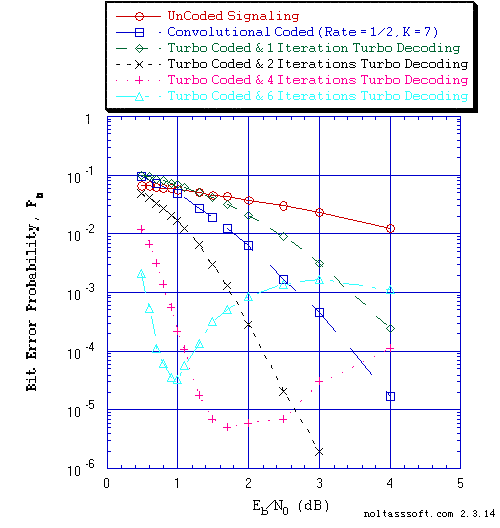```Figure 1. Bit Error Probability for UnCoded, Rate = 1/2 Convolutional Coded, and
Rate = 1/3 Turbo Coded (TC) BPSK Signaling over a Coherent Memoryless
Channel with Additive White Gaussian Noise (AWGN):
```
```          Equal probable Independent and Identical Distributed (IID) Source for
10 Million, 1 Million, and 1,001,472 Information Bits for UnCoded,
Convolutional Coded, and Turbo Coded BPSK Signaling respectively over
a Vector Channel;
```
```          Rate = 1/2, Constraint Length K = 7, (3,1,0,3,3,2,3), a Best (Optimal)
Non-Recursive Convolutional Code (J.P. Odenwalder) and Viterbi Algorithm
using a Path Memory Length of 35 bits and an Unquantized Branch Metric;
```
```          Rate (r) = 1/3, K = 3 Turbo Code based on a r = 1/2, K = 3 (G0 = 7 octal,
G1 = 5 octal) Recursive Systematic Convolutional component code and a
6144-Bit QPP Turbo Encoder Interleaver; and
```
```          Log-MAP Iterative Turbo Decoder using Systematic Channel Output as input
to both component decoders and Decode Information Bit Decision is based on
the Sum of both decoders' log-likelihood ratios (L-values) and for One
Iteration, Two Iterations, Four Iterations, and Six Iterations.
```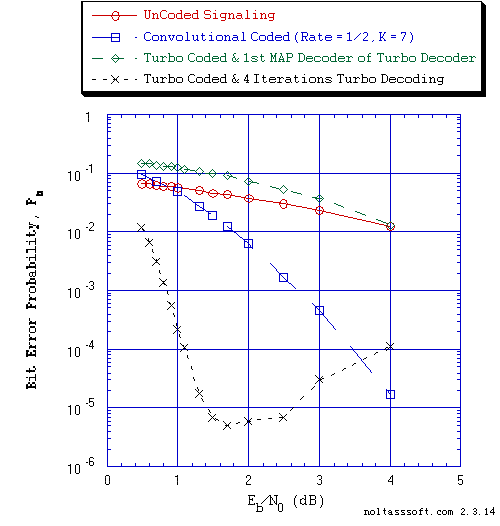```Figure 2. Bit Error Probability for UnCoded, Rate = 1/2 Convolutional Coded, and
Rate = 1/3 Turbo Coded (TC) BPSK Signaling over a Coherent Memoryless
Channel with Additive White Gaussian Noise (AWGN):
```
```          Equal probable Independent and Identical Distributed (IID) Source for
10 Million, 1 Million, and 1,001,472 Information Bits for UnCoded,
Convolutional Coded, and Turbo Coded BPSK Signaling respectively over
a Vector Channel;
```
```          Rate = 1/2, Constraint Length K = 7, (3,1,0,3,3,2,3), a Best (Optimal)
Non-Recursive Convolutional Code (J.P. Odenwalder) and Viterbi Algorithm
using a Path Memory Length of 35 bits and an Unquantized Branch Metric;
```
```          Rate (r) = 1/3, K = 3 Turbo Code based on a r = 1/2, K = 3 (G0 = 7 octal,
G1 = 5 octal) Recursive Systematic Convolutional component code and a
6144-Bit QPP Turbo Encoder Interleaver; and
```
```          Log-MAP Iterative Turbo Decoder using Systematic Channel Output as input
to both component decoders and Decode Information Bit Decision is based on
the Sum of both decoders' log-likelihood ratios (L-values) and for Four
Iterations.
```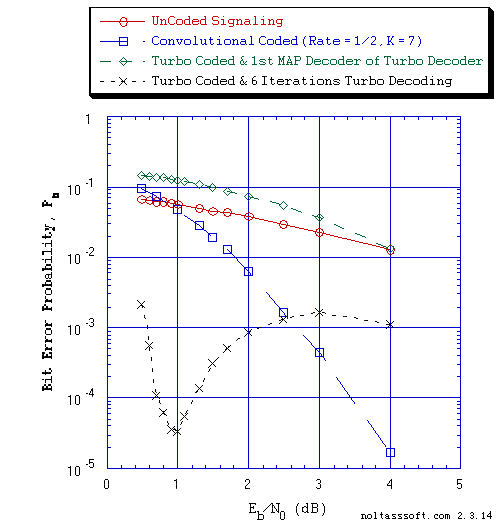```Figure 3. Bit Error Probability for UnCoded, Rate = 1/2 Convolutional Coded, and
Rate = 1/3 Turbo Coded (TC) BPSK Signaling over a Coherent Memoryless
Channel with Additive White Gaussian Noise (AWGN):
```
```          Equal probable Independent and Identical Distributed (IID) Source for
10 Million, 1 Million, and 1,001,472 Information Bits for UnCoded,
Convolutional Coded, and Turbo Coded BPSK Signaling respectively over
a Vector Channel;
```
```          Rate = 1/2, Constraint Length K = 7, (3,1,0,3,3,2,3), a Best (Optimal)
Non-Recursive Convolutional Code (J.P. Odenwalder) and Viterbi Algorithm
using a Path Memory Length of 35 bits and an Unquantized Branch Metric;
```
```          Rate (r) = 1/3, K = 3 Turbo Code based on a r = 1/2, K = 3 (G0 = 7 octal,
G1 = 5 octal) Recursive Systematic Convolutional component code and a
6144-Bit QPP Turbo Encoder Interleaver; and
```
```          Log-MAP Iterative Turbo Decoder using Systematic Channel Output as input
to both component decoders and Decode Information Bit Decision is based on
the Sum of both decoders' log-likelihood ratios (L-values) and for Six
Iterations.
```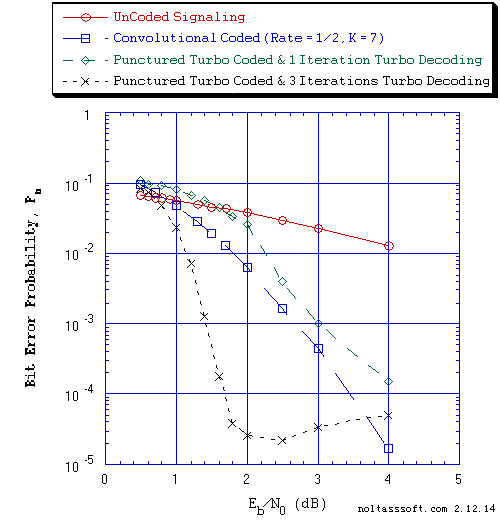```Figure 4. Bit Error Probability for UnCoded, Rate = 1/2 Convolutional Coded, and
Rate = 1/2 Punctured Turbo Coded (PTC) BPSK Signaling over a Coherent
Memoryless Channel with Additive White Gaussian Noise (AWGN):
```
```          Equal probable Independent and Identical Distributed (IID) Source for
10 Million, 1 Million, and 1,001,472 Information Bits for UnCoded,
Convolutional Coded, and PTC BPSK Signaling respectively over a Vector
Channel;
```
```          Rate = 1/2, Constraint Length K = 7, (3,1,0,3,3,2,3), a Best (Optimal)
Non-Recursive Convolutional Code (J.P. Odenwalder) and Viterbi Algorithm
using a Path Memory Length of 35 bits and an Unquantized Branch Metric;
```
```          Rate (r) = 1/5, K = 4 Parent Turbo Code based on a r = 1/3, K = 4 (G0 = 13
octal, G1 = 15 octal, G2 = 17 octal) Recursive Systematic Convolutional
component code (Optimum-weight Spectrum) and a 6144-Bit QPP Turbo Encoder
Interleaver;
```
```          Punctured Turbo Code Rate = 1/2 for Puncturing Period of 2 and Puncturing
Matrix = [[1,1],[1,0],[0,0][0,1],[0,0]]; and
```
```          Log-MAP Iterative Turbo Decoder using Systematic Channel Output as input
to both component decoders and Decode Information Bit Decision is based on
the Sum of both decoders' log-likelihood ratios (L-values) and for One and
Three Iterations.
```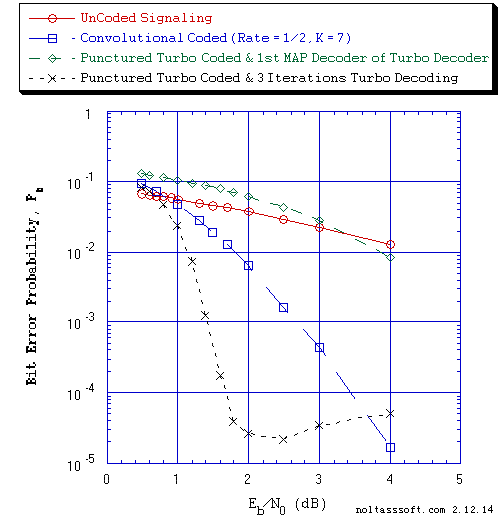```Figure 5. Bit Error Probability for UnCoded, Rate = 1/2 Convolutional Coded, and
Rate = 1/2 Punctured Turbo Coded (PTC) BPSK Signaling over a Coherent
Memoryless Channel with Additive White Gaussian Noise (AWGN):
```
```          Equal probable Independent and Identical Distributed (IID) Source for
10 Million, 1 Million, and 1,001,472 Information Bits for UnCoded,
Convolutional Coded, and PTC BPSK Signaling respectively over a Vector
Channel;
```
```          Rate = 1/2, Constraint Length K = 7, (3,1,0,3,3,2,3), a Best (Optimal)
Non-Recursive Convolutional Code (J.P. Odenwalder) and Viterbi Algorithm
using a Path Memory Length of 35 bits and an Unquantized Branch Metric;
```
```          Rate (r) = 1/5, K = 4 Parent Turbo Code based on a r = 1/3, K = 4 (G0 = 13
octal, G1 = 15 octal, G2 = 17 octal) Recursive Systematic Convolutional
component code (Optimum-weight Spectrum) and a 6144-Bit QPP Turbo Encoder
Interleaver;
```
```          Punctured Turbo Code Rate = 1/2 for Puncturing Period of 2 and Puncturing
Matrix = [[1,1],[1,0],[0,0][0,1],[0,0]]; and
```
```          Log-MAP Iterative Turbo Decoder using Systematic Channel Output as input
to both component decoders and Decode Information Bit Decision is based on
the Sum of both decoders' log-likelihood ratios (L-values) and for Three
Iterations.
```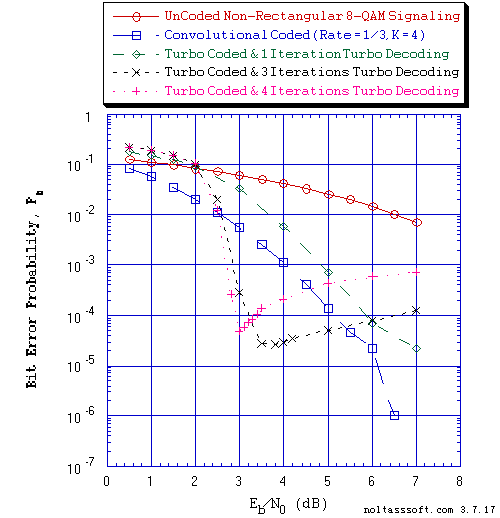```Figure 6. Bit Error Probability for UnCoded, Rate = 1/3 Convolutional Coded, and
Rate = 1/3 Turbo Coded (TC) Non-Rectangular 8-QAM Signaling over a
Coherent Memoryless Channel with Additive White Gaussian Noise (AWGN):
```
```          Equal probable Independent and Identical Distributed (IID) Source
for 10,000,002, 1 Million, and 1,001,472 Information Bits for UnCoded,
Convolutional Coded, and Turbo Coded Non-Rectangular (NR) 8-QAM Signaling
respectively over a Vector Channel;
```
```          Rate = 1/3, Constraint Length K = 4, (7,5,6,7), a Best (Optimal)
Non-Recursive Convolutional Code (J.P. Odenwalder) and Viterbi
Algorithm using a Path Memory Length of 20 bits and an Unquantized Branch
Metric;
```
```          Rate (r) = 1/3, K = 4 Turbo Code based on a r = 1/2, K = 4 (G0 = 13
octal, G1 = 17 octal) Recursive Systematic Convolutional component code
(Optimum-weight Spectrum) and a 6144-Bit QPP Turbo Encoder Interleaver;
```
```          NR 8-QAM Demodulation Constellation DeMapper Algorithm: Max-Log Bit
Metrics; and
```
```          Max-Log-MAP Iterative Turbo Decoder using Systematic Channel Output
as input to both component decoders and Decode Information Bit Decision
is based on the Sum of both decoders' log-likelihood ratios (L-values)
and for One Iteration, Three Iterations, and Four Iterations.
```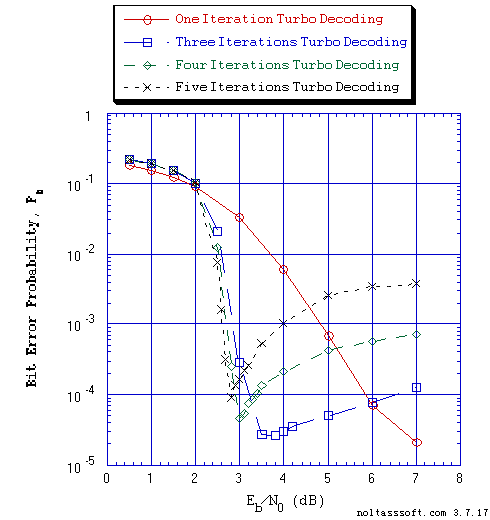```Figure 7. Bit Error Probability for Rate = 1/3 Turbo Coded (TC) Non-Rectangular (NR)
8-QAM Signaling over a Coherent Memoryless Channel with Additive
White Gaussian Noise (AWGN):
```
```          Equal probable Independent and Identical Distributed (IID) Source
for 1,001,472 Information Bits for Turbo Coded NR 8-QAM Signaling over
a Vector Channel;
```
```          Rate (r) = 1/3, K = 4 Turbo Code based on a r = 1/2, K = 4 (G0 = 13 octal,
G1 = 17 octal) Recursive Systematic Convolutional component code
(Optimum-weight Spectrum) and a 6144-Bit QPP Turbo Encoder Interleaver;
```
```          NR 8-QAM Demodulation Constellation DeMapper Algorithm: Max-Log Bit
Metrics; and
```
```          Max-Log-MAP Iterative Turbo Decoder using Systematic Channel
Output as input to both component decoders and Decode Information Bit
Decision is based on the Sum of both decoders' log-likelihood ratios
(L-values) and for One Iteration, Three Iterations, Four Iterations, and
Five Iterations.
```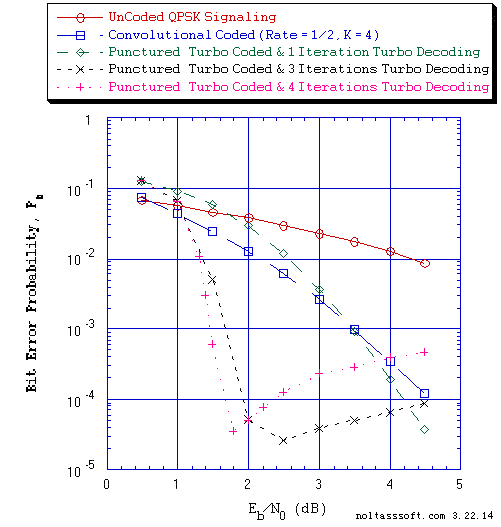```Figure 8. Bit Error Probability for UnCoded, Rate = 1/2 Convolutional Coded, and
Rate = 1/2 Punctured Turbo Coded (PTC) Gray Coded QPSK Signaling
over a Coherent Memoryless Channel with Additive White Gaussian Noise
(AWGN):
```
```          Equal probable Independent and Identical Distributed (IID) Source
for 10 Million, 1 Million, and 1,001,472 Information Bits for UnCoded,
Convolutional Coded, and PTC Gray Coded (GC) QPSK Signaling respectively
over a Vector Channel;
```
```          Rate = 1/2, Constraint Length K = 4, (3,2,3,3), a Best (Optimal)
Non-Recursive Convolutional Code (J.P. Odenwalder) and Viterbi Algorithm
using a Path Memory Length of 20 bits and an Unquantized Branch Metric;
```
```          Rate (r) = 1/3, K = 4 Parent Turbo Code based on a r = 1/2, K = 4 (G0 = 13
octal, G1 = 17 octal) Recursive Systematic Convolutional component code
(Optimum-weight Spectrum) and a 6144-Bit QPP Turbo Encoder Interleaver;
```
```          Punctured Turbo Code Rate = 1/2 for Puncturing Period of 2 and Puncturing
Matrix = [[1,1],[1,0],[0,1]];
```
```          GC QPSK Demodulation Constellation DeMapper Algorithm: Max-Log Bit
Metrics; and
```
```          Max-Log-MAP Iterative Turbo Decoder using Systematic Channel Output
as input to both component decoders and Decoder Information Bit Decision
is based on the Sum of both decoders' log-likelihood ratios (L-values)
and for One Iteration, Three Iterations, and Four Iterations.
```
References (Turbo Coding & Binary Modulation):

 Claude Berrou, Alain Glavieux, and Punya Thitimajshima, "Near Shannon Limit Error-Correcting Coding: Turbo -Codes (1)," in Proc., 1993 IEEE International Conference on Communications (Geneva, Switzerland), pp. 1064-1070, May 1993.

 Patrick Robertson, "Illuminating the Structure of Code and Decoder of Parallel Concatenated Recursive Systematic (Turbo) Codes," in Proc., IEEE Globecom Conference (San Francisco, CA), pp. 1296-1303, December 1994.

 D. Divsalar and F. Pollara, "Turbo Codes for PCS Applications," in Proc. IEEE ICC'95, Seattle, WA pp. 54-59, June 1995.

 Patrick Robertson, Emmanuelle Villebrun, and Peter Hoeher, "A Comparison of Optimal and Sub-Optimal MAP Decoding Algorithms Operating in the Log Domain," in Proc. IEEE ICC'95, Seattle, WA, pp. 1009-1013, June 1995.

 Sergio Benedetto and Guido Montorsi, "Unveiling Turbo Codes: Some Results on Parallel Concatenated Coding Schemes," IEEE Transactions on Information Theory, Vol. 42, No. 2, pp. 409-428, March 1996.

 Joachim Hagenauer, Elke Offer, and Lutz Papke, "Iterative Decoding of Binary Block and Convolutional Codes," IEEE Transactions on Information Theory, Vol. 42, No. 2, pp. 429-445, March 1996.

 Sergio Benedetto and Guido Montorsi, "Design of Parallel Concatenated Convolutional Codes," IEEE Transactions on Communications, Vol. 44, No. 5, pp. 591-600, May 1996.

 Claude Berrou and Alain Glavieux, "Near Optimum Error Correcting Coding and Decoding: Turbo Codes," IEEE Transactions on Communications, Vol. 44, No. 10, pp. 1261-1271, October 1996.

 Lance C. Perez, Jan Seghers, and Daniel J. Costello, Jr., "A Distance Spectrum Interpretation of Turbo Codes," IEEE Transactions on Information Theory, Vol. 42, No. 6, pp. 1698-1709, November 1996.

 A.J Viterbi, A.M. Viterbi, and N.T. Sindhushayana, "Interleaved concatenated codes: New perspective on approaching the Shannon limit," in Proc., Natl. Acad. Sci. USA, Vol. 94, pp. 9525-9531, September 1997.

 Sergio Benedetto, Roberto Garello, and Guido Montorsi, "A Search for Good Convolutional Codes to be Used in the Construction of Turbo Codes," IEEE Transactions on Communications, Vol. 46, No. 9, pp. 1101-1105, September 1998.

 Omer F. Acikel and William E. Ryan, " Punctured Turbo-Codes for BPSK/QPSK Channels," IEEE Transactions on Communications, Vol. 47, No. 9, pp. 1315-1323, September 1999.

 Roberto Garello, Paola Pierleoni, and Sergio Benedetto, "Computing the Free Distance of Turbo Codes and Serially Concatenated Codes with Interleavers: Algorithms and Applications," IEEE Journal on Selected Areas in Communications, Vol. 19, No. 5, pp. 800-812, May 2001.

 Shu Lin and Daniel J. Costello, Jr., Error Control Coding: fundamentals and applications, Second Edition, Pearson Prentice Hall, Upper Saddle River, New Jersey, 2004.

 Jing Sun and Oscar Y. Takeshita, "Interleavers for Turbo Codes Using Permutation Polynomials over Integer Rings," IEEE Transactions on Information Theory, Vol. 51, No. 1, pp. 101-119, January 2005.

 Oscar Y. Takeshita, "On Maximum Contention-Free Interleavers and Permutation Polynomials over Integer Rings," IEEE Transactions on Information Theory, Vol. 52, No. 3, pp. 1249-1253, March 2006.

 Guang-Chong Zhu, and Fady Alajaji, "Joint Source-Channel Turbo Coding for Binary Markov Sources," IEEE Transactions on Wireless Communications, Vol. 5, No. 5, pp. 1065-1075, May 2006.

 Oscar Y. Takeshita, "Permutation Polynomial Interleavers: An Algebraic-Geometric Perspective," IEEE Transactions on Information Theory, Vol. 53, No. 6, pp. 2116-2132, June 2007.

 Yang Sun and Joseph R. Cavallaro, "Efficient hardware implementation of a highly-parallel 3GPP LTE/LTE-advance turbo decoder," INTEGRATION, the VLSI journal, Vol. 44, pp. 305-315, 2011.

References (Turbo Coding & M > 2 Modulation):

 Stephane Le Goff, Alain Glavieux, and Claude Berrou, "Turbo-Codes and High Spectral Efficiency Modulation," in Proc. of 1994 IEEE International Conference on Communications (New Orleans, LA), ICC '94, pp. 645-649, May 1994.

 Patrick Robertson and Thomas Worz, "A Novel Bandwidth Efficient Coding Scheme Employing Turbo Codes," in Proc. of 1996 IEEE International Conference on Communications (Dallas, TX), ICC '96, pp. 962-967, June 1996.

 Filippo Tosato and Paola Bisaglia, "Simplified Soft-Output Demapper for Binary Interleaved COFDM with Application to HIPERLAN/2," HP Technical Report HPL-2001-246, October 10, 2001; or in Proc. IEEE International Conference on Communications, 2002, ICC 2002, Vol. 2, pp. 664-668, 2002.

 Ramesh Pyndiah, Annie Picart, and Alain Glavieus, "Performance of Block Turbo Coded 16-QAM and 64-QAM Modulations," in Proc. of IEEE GLOBECOM '95, pp. 1039-1043, November 1995.

 Ramesh Pynadiah, Alain Glavieus, Annie Picart, and Sylvie Jacq, "Near Optimum Decoding of Product Codes," in Proc. of IEEE GLOBECOM '94, pp. 339-343, November - December 1994.

 Ephraim Zehavi, "8-PSK Trellis Codes for a Rayleigh Channel", IEEE Transactions on Communications, Vol. 40, No. 5, pp. 873-884, May 1992.

 Lifang Li, Dariush Divsalar, and Samuel Dolinar, "Iterative Demodulation, Demapping, and Decoding of Coded Non-Square QAM," IEEE Transactions on Communications, Vol. 53, No. 1, pp. 16-19, January 2005.

 Keunhyung Lee, Donghoon Kang, Hyobae Park, and Wangrok Oh, "Joint Encoding and Modulation Scheme for Binary Turbo Code and 16-QAM," The 6th International Conference on Information Technology and Applications (ICITA 2009), pp. 307-310, 2009.

```BUY T1 Version 2 (ADVDCSMT1DCSS Professional software system tool)NOW.
```